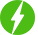# Numerical Techniques for Solution of Transcendental Equation

1

Numerical Techniques for Solution of Transcendental Equation, Solution of Transcendental equation.

## Course Description

With availability of digital computers it is simple to solve completed problems based on mathematics. Numerical methods are extremely powerful problem solving tools. They are capable of handling large systems of equations, nonlinearities and complicated geometries that are uncommon in engineering practice and often impossible to solve by traditional ways. Numerical methods enhance your problem solving skills. Transcendental equations are those which consist of terms like trigonometric, logarithmic and exponential terms. Solving such equation by traditional ways is time consuming and these are not solved with the help of digital computers.

A numerical method gives very simple ways to enhance your understanding of mathematics. Because one function of numerical methods is to reduce higher mathematics to basic arithmetic operations. Numerical methods can be implemented by writing algorithm and implanting these steps by using any language in the digital computers.

This course is a very basic for studying numerical methods for transcendental equations.

In this course we will study concept of root, Intermediate value theorem and bracketing and open ended methods to solve transcendental equations with graphical explanation. Also which methods converges fast is explained by solving one numerical by all methods. At the end comparison of all methods is done with various parameters.

Free
We will be happy to hear your thoughtsRegister New Account
• Total (0)
0